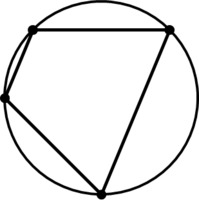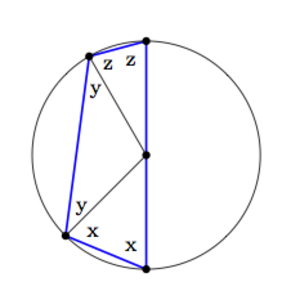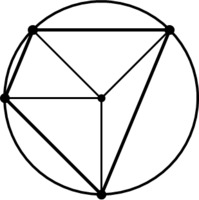# Opposite Angles in a Cyclic Quadrilateral

Alignments to Content Standards: G-C.A.3

A cyclic quadrilateral is a quadrilateral whose vertices all lie on a circle. An example is pictured below:Prove that the opposite angles in a cyclic quadrilateral that contains the center of the circle are supplementary.

## IM Commentary

The goal of this task is to show that opposite angles in a cyclic quadrilateral are supplementary. The converse of this result is also true and was established by Euclid: http://en.wikipedia.org/wiki/Cyclic_quadrilateral. Students will need plenty of time to work on this task since, similar to the result showing that a triangle inscribed in a semicircle is a right triangle, they must produce auxiliary radii of the circle and then patiently manipulate the resulting equations for the different angles.

In the given picture, the inscribed quadrilateral contains the circle's center. The argument relies upon this fact and different arguments are required to deal with the cases where the center of the circle lies on the quadrilateral and where the center of the circle is outside of the quadrilateral. For example, the picture below shows the case where one side of the quadrilateral is a diameter:In this case the argument for why opposite angles are supplementary is simpler as the opposite angles have measures $x$ and $y + z$ for one pair and $x+y$ and $z$ for the other. So we need to establish that $x+y+z = 180$ which is true because $2x+2y+2z = 360$.

The third case, where the center of the circle lies outside the quadrilateral, is the most complex but could be given to advanced students as a challenge problem. If techonology is available, students may wish to experiment with different quadrilaterals to see how the angles vary. The teacher may wish to do this activity first as the students might be led to conjecture that opposite angles in a cyclic quadrilateral are supplementary and thereby be ready and motivated to work on this task.

## Solution

Construct a radius to each of the four vertices of the quadrilateral as pictured below:Since the radii of the circle are all congruent, this partitions the quadrilateral into four isosceles triangles. The base angles of an isosceles triangle have the same measure. These pairs of congruent angles are labeled in the picture below:The sum of the angles around the center of the circle is 360 degrees. The sum of the angles in each of the triangles is 180 degrees. So if we add up the labeled angle measures and the angles forming the circle around the center center, we get: $$2a+2b+2c+2d+360=4\cdot 180.$$ If we subtract 360 from both sides, we get $$2a+2b+2c+2d=360$$ or $$a+b+c+d = 180.$$ Alternatively, we may use the fact that the sum of angles in a quadrilateral is 360 degrees and bypass the argument with the angles making a circle.

Note that $(a+b)$ and $(c+d)$ are the measures of opposite angles, and we can simply group the measures in the last equation like this: $$(a+b)+(c+d) = 180.$$ Likewise, $(a+d)$ and $(b+c)$ are the measures of opposite angles, and we can just rearrange the equation to see that $$(a+d)+(b+c) = 180.$$ So, indeed, we see that the opposite angles in a cyclic quadrilateral are supplementary.